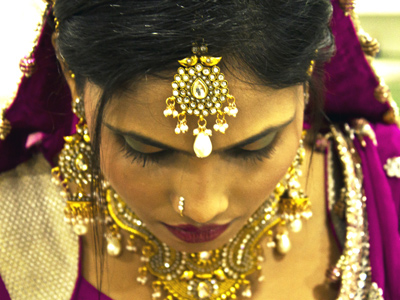Question 7 asks if some gold jewellery is real or not. Can you perform the calculation required?

# Conversion Problems (Metric to Metric) (F)

So, no imperial measures in this GCSE Maths metric conversion quiz, did you think you were safe? Think again! The metric system is based around a standard base unit (called SI, or Systeme International) for each type of measurement – the metre for length, gram for mass, and litre for volume.

If we want to describe very large or very small measurements, we need to add a prefix to the base unit. You will recognise many of these:

• milli means thousandth (1mm is one thousandth of a metre, or there are 1,000mm in 1m)
• kilo means thousand, so a kilogram is 1,000 grams
• centi, as in centimetre, is one hundredth

Do you know any others? You should be very familiar working between the measures that are common in everyday life.

Volume is the measure that probably causes most problems, particularly when it involves liquids. A key fact to remember is that 1 litre of water weighs 1kg, and a cubic metre is really big! Mark it out on the floor, 1 metre by 1 metre square, then raise it up by a metre. If this cube were filled with water, it would weight a ton (metric of course!).

Question 1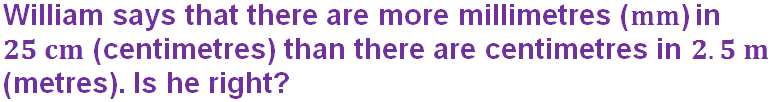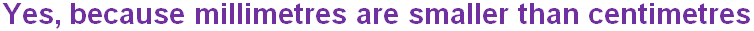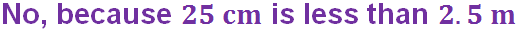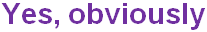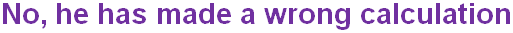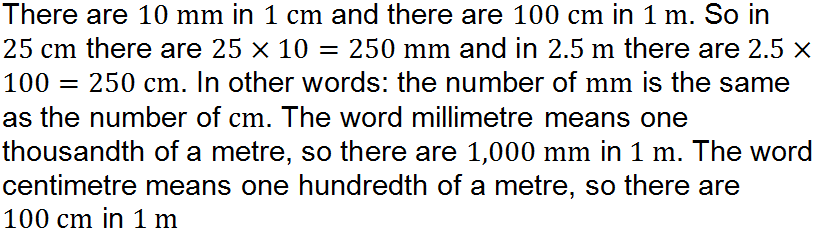Question 2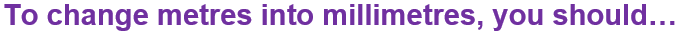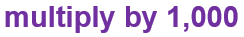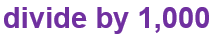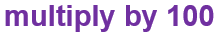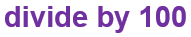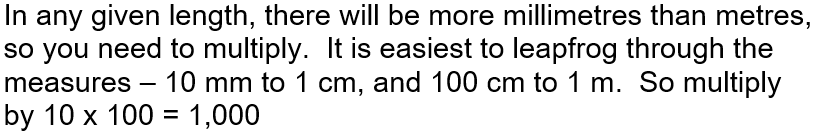Question 3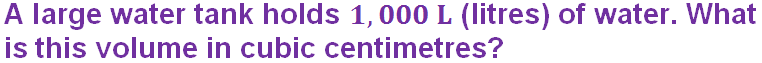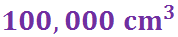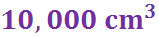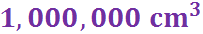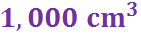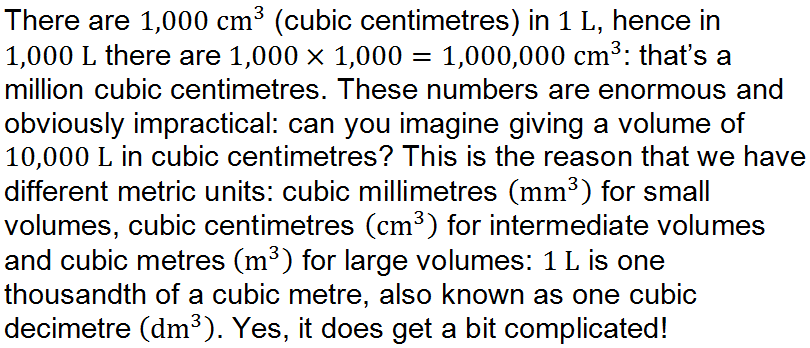Question 4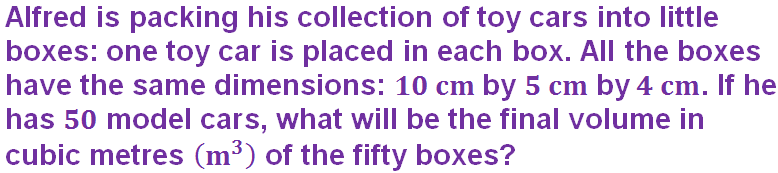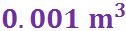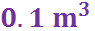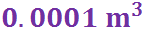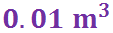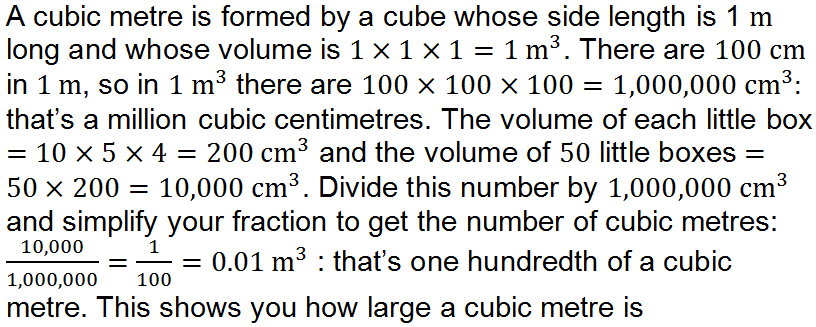Question 5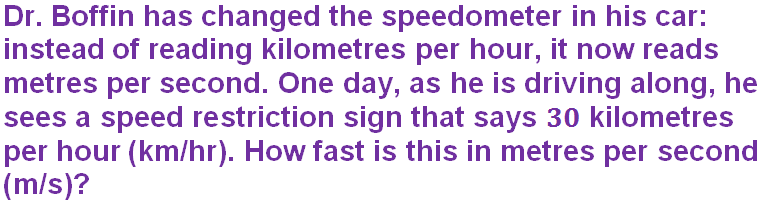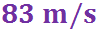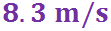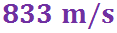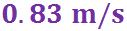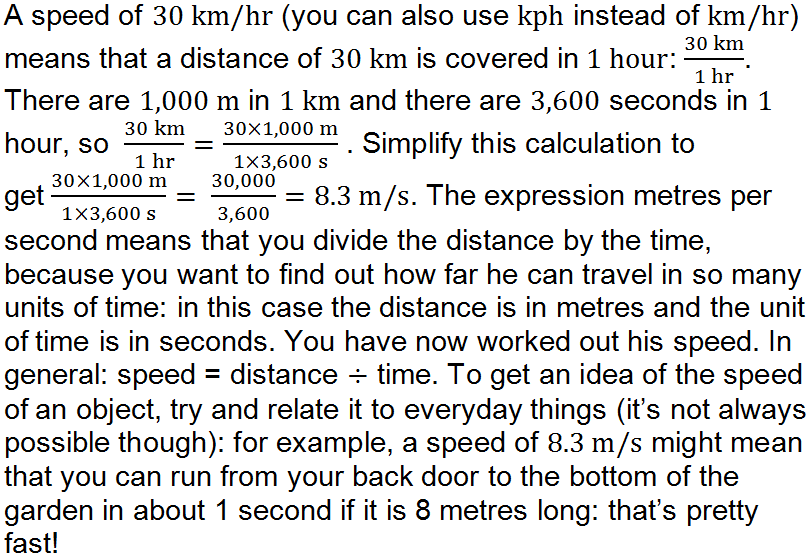Question 6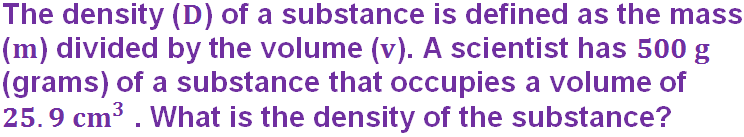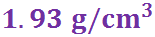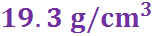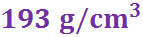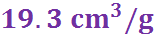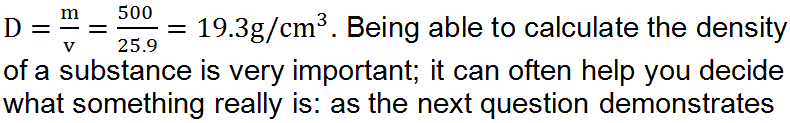Question 7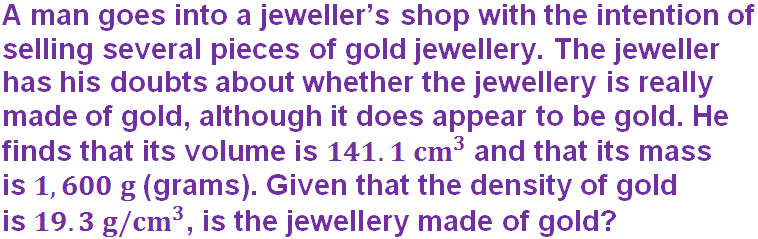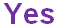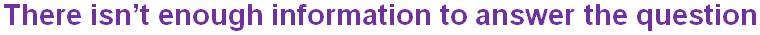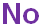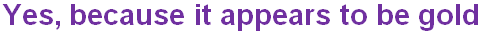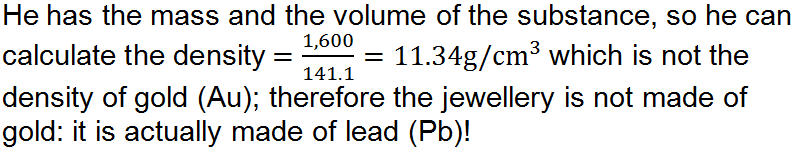Question 8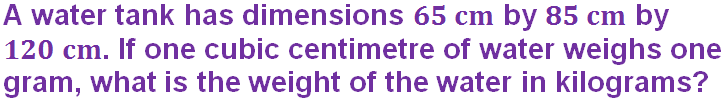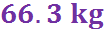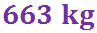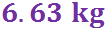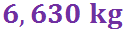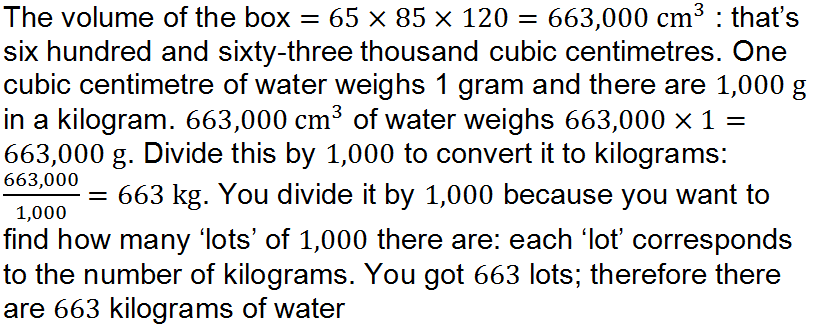Question 9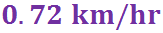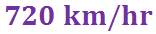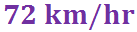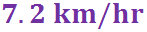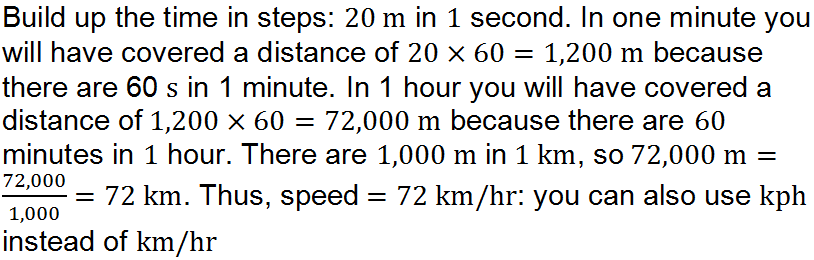Question 10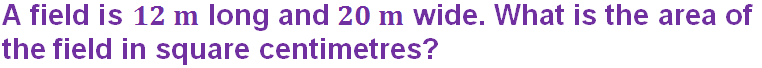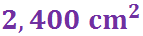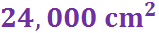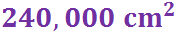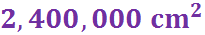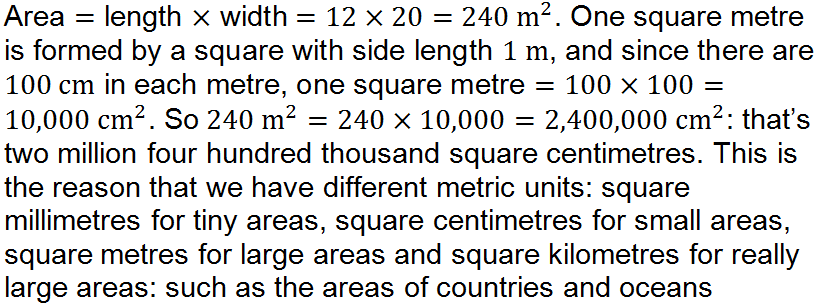Author:  Frank Evans